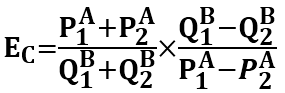# Cross-Price Elasticity of Demand (Ec-PED) Calculation(Step by step calculation)#Cross-Price Elasticity of Demand (Ec) Calculator

Ec measures the demand of Product A when price of unrelated Product B is changed.

Step by step calculation

#### Cross-Price Elasticity of the Demand Formula 1

Cross-Price Elasticity of the Demand (Ec) = P1 / P2

Where,

Ec is Cross-Price Elasticity of the Demand

P1 is Percent Change in a Quantity of Good A

P2 is Percent Change in the Price of Good B

Step by step calculation

#### Cross-Price Elasticity of the Demand Formula 2Where

Ec is the cross-price elasticity of the demand

P1A is the price of good A at time 1

P2A is the price of good A at time 2

Q1B is the quantity of good B at time 1

Q2B is the quantity of good B at time 2##Related Calculator

Straight-Line Depreciation Calculator##Age Calculators ►

Horse Age Calculator , Cow Age Calculator , Cat Age Calculator , Dog Age Calculator, Human Age Calculator 100 Days Old Calculator Date Difference Calculator